Want to share your content on R-bloggers? click here if you have a blog, or here if you don't.

Congratulations to Joshua Angrist and Guido Imbens for the Nobel Prize for their work with causality. This post will also be about causality, although not from the work of Angrist or Imbens. A year ago I read The Book of Why by Judea Pearl and Dana MacKenzie. The Book of Why states that you can make causal conclusions from observational data if you know the directed acyclical graph (DAG) of the processes that has created the data. The Book of Why also states that you can not create the DAG based on data alone, i.e. you can not have the data as an input to an algorithm and get the DAG and its causal implications as output. The Book of Why also explains that Judea Pearl has studied Bayesian networks before he began his work with DAGs and causality. I hypothesise that some DAGS are more probable than other DAGs based on the statistics of the data. I am examining if there are ways to get boundaries of the causal effects that variables can have on each other within a limited system. I will use structure learning algorithms for Bayesian networks. I will take no regard to unmeasured confounders. This work is ongoing and the results are as is. I will use data from Statistics Sweden, for more information about the data see my previous posts.

Statistics Sweden use NUTS (Nomenclature des Unités Territoriales Statistiques), which is the EU’s hierarchical regional division, to specify the regions.

First, define libraries and functions.

```library(tidyverse)
## -- Attaching packages --------------------------------------- tidyverse 1.3.1 --
## v ggplot2 3.3.5     v purrr   0.3.4
## v tibble  3.1.5     v dplyr   1.0.7
## v tidyr   1.1.4     v stringr 1.4.0
## v readr   2.0.2     v forcats 0.5.1
## Warning: package 'ggplot2' was built under R version 4.0.5
## Warning: package 'tibble' was built under R version 4.0.5
## Warning: package 'tidyr' was built under R version 4.0.5
## Warning: package 'readr' was built under R version 4.0.5
## Warning: package 'dplyr' was built under R version 4.0.5
## Warning: package 'forcats' was built under R version 4.0.3
## -- Conflicts ------------------------------------------ tidyverse_conflicts() --
library(gtools)
## Warning: package 'gtools' was built under R version 4.0.5
library(pcalg)
## Warning: package 'pcalg' was built under R version 4.0.5
library(imputeMissings)
## Warning: package 'imputeMissings' was built under R version 4.0.5
##
## Attaching package: 'imputeMissings'
## The following object is masked from 'package:dplyr':
##
##     compute
library(bnlearn)
## Warning: package 'bnlearn' was built under R version 4.0.5
##
## Attaching package: 'bnlearn'
## The following object is masked from 'package:imputeMissings':
##
##     impute
## The following objects are masked from 'package:pcalg':
##
##     dsep, pdag2dag, shd, skeleton
library(dagitty)
## Warning: package 'dagitty' was built under R version 4.0.4
##
## Attaching package: 'dagitty'
## The following objects are masked from 'package:bnlearn':
##
##     ancestors, children, descendants, parents, spouses
## The following object is masked from 'package:pcalg':
##
##     randomDAG
library(AER)
## Warning: package 'AER' was built under R version 4.0.5
## Warning: package 'car' was built under R version 4.0.5
## Warning: package 'carData' was built under R version 4.0.3
##
## Attaching package: 'car'
## The following object is masked from 'package:gtools':
##
##     logit
## The following object is masked from 'package:dplyr':
##
##     recode
## The following object is masked from 'package:purrr':
##
##     some
## Warning: package 'lmtest' was built under R version 4.0.4
## Warning: package 'zoo' was built under R version 4.0.5
##
## Attaching package: 'zoo'
## The following objects are masked from 'package:base':
##
##     as.Date, as.Date.numeric
## Warning: package 'sandwich' was built under R version 4.0.5
## Warning: package 'survival' was built under R version 4.0.5
library(lavaan)
## Warning: package 'lavaan' was built under R version 4.0.5
## This is lavaan 0.6-9
## lavaan is FREE software! Please report any bugs.
library(semPlot)
## Warning: package 'semPlot' was built under R version 4.0.3
library(psych)
## Warning: package 'psych' was built under R version 4.0.5
##
## Attaching package: 'psych'
## The following object is masked from 'package:lavaan':
##
##     cor2cov
## The following object is masked from 'package:car':
##
##     logit
## The following object is masked from 'package:gtools':
##
##     logit
## The following objects are masked from 'package:ggplot2':
##
##     %+%, alpha
readfile <- function (file1){read_csv (file1, col_types = cols(), locale = readr::locale (encoding = "latin1"), na = c("..", "NA")) %>%
gather (starts_with("19"), starts_with("20"), key = "year", value = groupsize) %>%
drop_na() %>%
mutate (year_n = parse_number (year))
}

perc_women <- function(x){
ifelse (length(x) == 2, x / (x + x), NA)
}

mutate(NUTS2_sh = substr(NUTS2, 3, 4))

nuts %>%
distinct (NUTS2_en) %>%
knitr::kable(
booktabs = TRUE,
caption = 'Nomenclature des Unités Territoriales Statistiques (NUTS)')```
Table 1: Nomenclature des Unités Territoriales Statistiques (NUTS)
NUTS2_en
SE11 Stockholm
SE12 East-Central Sweden
SE21 Småland and islands
SE22 South Sweden
SE23 West Sweden
SE31 North-Central Sweden
SE32 Central Norrland
SE33 Upper Norrland
```# normalize data
tonormest <- function(tablearg){
norm_recipe <- recipes::recipe( ~ ., data = tablearg) %>%
recipes::step_normalize(recipes::all_numeric())

prepare <- recipes::prep(norm_recipe, training = tablearg)

return (recipes::bake(prepare, new_data = tablearg))
}

# not in set
`%nin%` = Negate(`%in%`)

# create a set of arcs not allowed in model
createblacklist <- function(coln, exogenous, endogenous = NULL){
rbind(
expand.grid(endogenous, coln[coln %nin% endogenous]),
expand.grid(coln[coln %nin% exogenous], exogenous))
}

# list of models in bnlearn to evaluate
bnmodels <- list(
h2pc = function(x, blacklist = blacklist) bnlearn::h2pc(x, blacklist = blacklist),
hpc = function(x, blacklist = blacklist) bnlearn::hpc(x, blacklist = blacklist),
fast.iamb = function(x, blacklist = blacklist) bnlearn::fast.iamb(x, blacklist = blacklist),
inter.iamb = function(x, blacklist = blacklist) bnlearn::inter.iamb(x, blacklist = blacklist),
si.hiton.pc = function(x, blacklist = blacklist) bnlearn::si.hiton.pc(x, blacklist = blacklist),
iamb.fdr = function(x, blacklist = blacklist) bnlearn::iamb.fdr(x, blacklist = blacklist),
iamb = function(x, blacklist = blacklist) bnlearn::iamb(x, blacklist = blacklist),
gs = function(x, blacklist = blacklist) bnlearn::gs(x, blacklist = blacklist),
mmpc = function(x, blacklist = blacklist) bnlearn::mmpc(x, blacklist = blacklist),
pc = function(x, blacklist = blacklist) bnlearn::pc.stable(x, blacklist = blacklist),
hc = function(x, blacklist = blacklist) bnlearn::hc(x, blacklist = blacklist),
tabu = function(x, blacklist = blacklist) bnlearn::tabu(x, blacklist = blacklist),
mmhc = function(x, blacklist = blacklist) bnlearn::mmhc(x, blacklist = blacklist),
rsmax2 = function(x, blacklist = blacklist) bnlearn::rsmax2(x, blacklist = blacklist))

# evaluate all models on a dataset given a blacklist
evaluatemodel <- function(tablearg, blacklist){
evalslfunc <- function(f){
sltree <- f (data.frame(map(tablearg, inttonumeric)), blacklist = blacklist)
neltree <- as.graphNEL(sltree)
if (length(bg) == 0){return (Inf)}
g <- dagitty(pcalg2dagitty(t(as(bg, "matrix")), colnames(tablearg), type = "dag"))
r <- localTests(
g,
tablearg, "cis",
sample.cov = lavCor(tablearg),
sample.nobs = nrow( tablearg ),
max.conditioning.variables = 2,
R = 100)
if (dim(r) == 0){return (0)}
return(mean(abs(r\$estimate)))
}

return(data.frame(lapply(bnmodels, evalslfunc)))
}

# Change all variables of integer type to a numeric type
inttonumeric <- function(x){
if(is.integer(x)){
return(as.numeric(x))
}
else{
return(x)
}
}

# create a structured model from a dataset and a structured learning algorithm
createmodel <- function(tablearg, f, blacklist){
sltree <- f (data.frame(map(tablearg, inttonumeric)), blacklist = blacklist)
neltree <- as.graphNEL(sltree)
g <- dagitty(pcalg2dagitty(t(as(bg, "matrix")), colnames(tablearg), type = "dag"))

return(g)
}

# change the sign from dagitty syntax to lavaan syntax
changesign <- function(s){
if(s == "->"){
return("~")
}
if (s == "--"){
return("~~")
}
}

# create a list of all combinations of variables, one variable from each factor from the factor analysis, and subsets thereof
allcombn <- function(gridarg){
allcombn_worker <- function(gridarg){
sumcomb <- vector()
for(i in data.frame(t(gridarg))){
subcomb <- combn(i, length(gridarg) - 1)
for(j in data.frame(subcomb)){
sumcomb <- rbind(sumcomb, j)
}
}
return(unique(sumcomb))
}

sumcomb <- list()
sumcomb <- append(sumcomb, split(gridarg, seq(nrow(gridarg))))
subcomb <- allcombn_worker(gridarg)
if(length(data.frame(subcomb)) > 0){
sumcomb <- append(sumcomb, allcombn(data.frame(subcomb)))
}
return(sumcomb)
}

# convert a list of variables, use each list element as a blacklist, find the structured learning algorithm with the lowest deviance, use that algorithm and create a model
# return:
# model with the lowest deviance
# the deviances for the different models
# the name of the algorithm with the lowest deviance
combtodag <- function(mycomb, tablearg){
summary_table <- vector()
for(i in 1:length(mycomb)){
j <- unlist(mycomb[i])
blacklist <- createblacklist(colnames(tablearg), j)
evaluatedmodel <- evaluatemodel(tablearg, blacklist)
g <- createmodel(tablearg, match.fun(colnames(sort(evaluatedmodel)) ), blacklist)
v <- as.character(j)
length(v) <- 5
summary_table <- rbind(summary_table, c(as.character(g), t(evaluatedmodel), v,  colnames(sort(evaluatedmodel)) ))
}
return(summary_table)
}

# calculate the causal effect of the variable from to variable to using all models in listofmodels using all minimal adjustment sets that are given by the model
calceffect <- function(listofmodels, from, to, tablearg){
summary_table <- vector()
paste0(to, " ~ ", from, " + ", paste(unlist(adjsets), collapse = " + "))
}
calclmeffect <- function(eq){
(summary(lm(as.formula(eq), tablearg)) %>% broom::tidy())[2,]
}
for(i in 1:nrow(data.frame(listofmodels))){
g <- as.dagitty(listofmodels[i, 1])
exogenous <- listofmodels[i, 16:20]
eq <- paste(to, " ~ ",  from)
} else {
} else{
eq <- NULL
}
}

if (!is.null(eq)){
mytest <- map(unlist(eq), calclmeffect)
} else{
mytest <- NULL
}

v <- as.character(exogenous)
length(v) <- 5
v1 <- t(data.frame(v))
colnames(v1) <- c("1" , "2", "3", "4", "5")
if(!is.null(mytest)){
summary_table <- rbind(summary_table, cbind(Reduce('rbind', mytest), t(data.frame(eq)), v1))
}
}
return(summary_table)
}

# create an SEM model for each dagitty model and return a table containing the estimated parameters of the fitted model and a variety of fit measures for each model
dagitty2sem <- function(mycomb, tablearg){
summary_table <- vector()
summary_table2 <- vector()
for(i in 1:nrow(data.frame(mycomb))){
g <- as.dagitty(mycomb[i, 1])
exogenous <- mycomb[i, 16:20]
fit <- suppressWarnings(sem(paste(dagityy2lavaan(g, exogenous), collapse = ''), data = tablearg))
fit_m <- fitmeasures(fit)
v <- as.character(exogenous)
length(v) <- 5
sumfit <- parameterEstimates(fit)
summary_table2 <- rbind(summary_table2, cbind(sumfit, matrix(v, ncol = 5, nrow = nrow(sumfit), byrow = TRUE)))
summary_table <- rbind(summary_table, c(t(fit_m), v))
}

summary_table <- cbind(summary_table[,43:47], data.frame(map(data.frame(summary_table[,1:42]), as.numeric)))
colnames(summary_table) <- c(c("1" , "2", "3", "4", "5"), names(fit_m))

summary_table2 <- summary_table %>%
left_join(summary_table2, by = c("1" , "2", "3", "4", "5"))

return(summary_table2)
}

# convert a model with dagitty syntax to lavaan syntax. Relations between exogenous variables will be replaced by correlations
dagityy2lavaan <- function(model_arg, exogenous){
temp <- str_split(model_arg, "\n") %>%
unlist()
temp <- lapply("->|--", grep, x = temp, value = TRUE) %>%
unlist() %>%
data.frame() %>%
as_tibble()

colnames(temp) <- "data"

temp <- temp %>%
rowwise() %>%
mutate(lhs = unlist(str_split(data, " "))) %>%
mutate(rhs = unlist(str_split(data, " "))) %>%
mutate(sign = unlist(str_split(data, " ")))

# one variable can be exogenous but not both
endorel <-
temp %>% dplyr::filter(!(lhs %in% exogenous & rhs %in% exogenous))
exorel <- temp %>%
dplyr::filter(lhs %in% exogenous & rhs %in% exogenous)

exorel\$sign <- "--"

temp <- data.frame(rbind(endorel, exorel)) %>%
rowwise() %>% mutate(mydata3 = paste(rhs, changesign(sign), lhs, "\n"))

return(temp\$mydata3)
}

}```

The data tables are downloaded from Statistics Sweden. They are saved as a comma-delimited file without heading, http://www.statistikdatabasen.scb.se/pxweb/en/ssd/.

The tables:

UF0506A1_20210926-160849.csv: Population 16-74 years of age by region, highest level of education, age and sex. Year 1985 – 2020 NUTS 2 level 2008- 10 year intervals (16-74)

000000CG_20210926-160057.csv: Average basic salary, monthly salary and women´s salary as a percentage of men´s salary by region, sector, occupational group (SSYK 2012) and sex. Year 2014 – 2020 Monthly salary All sectors.

000000CD_20210926-160259.csv: Average basic salary, monthly salary and women´s salary as a percentage of men´s salary by region, sector, occupational group (SSYK 2012) and sex. Year 2014 – 2020 Number of employees All sectors.

The data is aggregated, the size of each group is in the column groupsize.

I have also included some calculated predictors from the original data.

nremployees: The number of employees in each group defined by ssyk, edulevel, region and year

perc_women: The percentage of women within each group defined by ssyk, edulevel, region and year

perc_women_region: The percentage of women within each group defined by ssyk, year and region

regioneduyears: The average number of education years per capita within each group defined by ssyk, year and region

eduquotient: The quotient between regioneduyears for men and women

salaryquotient: The quotient between salary for men and women within each group defined by ssyk, year and region

perc_women_ssyk_region: The percentage of women within each group defined by ssyk, year and region

```numedulevel <- read.csv("edulevel_1.csv")

numedulevel[, 2] <- data.frame(c(8, 9, 10, 12, 13, 15, 22, NA))

left_join(tb, by = c("region", "year", "sex", "sector","occuptional  (SSYK 2012)"))

right_join(tb, by = c("region", "year", "sex")) %>%
right_join(numedulevel, by = c("level of education" = "level.of.education")) %>%
filter(!is.na(eduyears)) %>%
mutate(edulevel = `level of education`) %>%
group_by(edulevel, region, year, sex, `occuptional  (SSYK 2012)`) %>%
mutate(groupsize_all_ages = sum(groupsize)) %>%
group_by(edulevel, region, year, `occuptional  (SSYK 2012)`) %>%
mutate (perc_women = perc_women (groupsize_all_ages[1:2])) %>%
mutate (nremployees = sum(groupsize.x)) %>%
mutate (salary = (groupsize.y * groupsize.x + groupsize.y * groupsize.x)/(groupsize.x + groupsize.x)) %>%
group_by (sex, year, region, `occuptional  (SSYK 2012)`) %>%
mutate(regioneduyears_sex = sum(groupsize * eduyears) / sum(groupsize)) %>%
mutate(regiongroupsize = sum(groupsize)) %>%
mutate(nremployees_sex = groupsize.x) %>%
group_by(region, year, `occuptional  (SSYK 2012)`) %>%
mutate (sum_pop = sum(groupsize)) %>%
mutate (regioneduyears = sum(groupsize * eduyears) / sum(groupsize)) %>%
mutate (perc_women_region = perc_women (regiongroupsize[1:2])) %>%
mutate (eduquotient = regioneduyears_sex / regioneduyears_sex) %>%
mutate (salary_sex = groupsize.y) %>%
mutate (salaryquotient = salary_sex / salary_sex) %>%
mutate (perc_women_ssyk_region = perc_women(nremployees_sex[1:2])) %>%
left_join(nuts %>% distinct (NUTS2_en, NUTS2_sh), by = c("region" = "NUTS2_en")) %>%
drop_na()

summary(tb)
##     region              age            level of education     sex
##  Length:316974      Length:316974      Length:316974      Length:316974
##  Class :character   Class :character   Class :character   Class :character
##  Mode  :character   Mode  :character   Mode  :character   Mode  :character
##
##
##
##      year             groupsize         year_n        sector
##  Length:316974      Min.   :    0   Min.   :2014   Length:316974
##  Class :character   1st Qu.: 2561   1st Qu.:2015   Class :character
##  Mode  :character   Median : 7456   Median :2017   Mode  :character
##                     Mean   :11676   Mean   :2017
##                     3rd Qu.:16443   3rd Qu.:2019
##                     Max.   :81358   Max.   :2020
##  occuptional  (SSYK 2012)  groupsize.x       year_n.x     groupsize.y
##  Length:316974            Min.   :  100   Min.   :2014   Min.   : 20200
##  Class :character         1st Qu.:  400   1st Qu.:2015   1st Qu.: 29100
##  Mode  :character         Median : 1100   Median :2017   Median : 34300
##                           Mean   : 2893   Mean   :2017   Mean   : 37470
##                           3rd Qu.: 3000   3rd Qu.:2019   3rd Qu.: 42600
##                           Max.   :53700   Max.   :2020   Max.   :139500
##     year_n.y       eduyears       edulevel         groupsize_all_ages
##  Min.   :2014   Min.   : 8.00   Length:316974      Min.   :   405
##  1st Qu.:2015   1st Qu.: 9.00   Class :character   1st Qu.: 24027
##  Median :2017   Median :12.00   Mode  :character   Median : 56038
##  Mean   :2017   Mean   :12.71                      Mean   : 70055
##  3rd Qu.:2019   3rd Qu.:15.00                      3rd Qu.:111943
##  Max.   :2020   Max.   :22.00                      Max.   :288426
##    perc_women      nremployees         salary       regioneduyears_sex
##  Min.   :0.3575   Min.   :   600   Min.   : 20200   Min.   :11.18
##  1st Qu.:0.4439   1st Qu.:  4800   1st Qu.: 29214   1st Qu.:11.65
##  Median :0.4816   Median : 13080   Median : 34488   Median :11.83
##  Mean   :0.4831   Mean   : 32797   Mean   : 37494   Mean   :11.86
##  3rd Qu.:0.5000   3rd Qu.: 37800   3rd Qu.: 42738   3rd Qu.:12.13
##  Max.   :0.6484   Max.   :426600   Max.   :123796   Max.   :12.64
##  regiongroupsize  nremployees_sex    sum_pop        regioneduyears
##  Min.   :127118   Min.   :  100   Min.   : 127118   Min.   :11.18
##  1st Qu.:291940   1st Qu.:  400   1st Qu.: 518853   1st Qu.:11.63
##  Median :528643   Median : 1100   Median : 722010   Median :11.85
##  Mean   :490383   Mean   : 2893   Mean   : 878571   Mean   :11.86
##  3rd Qu.:708813   3rd Qu.: 3000   3rd Qu.:1395157   3rd Qu.:12.01
##  Max.   :842459   Max.   :53700   Max.   :1682100   Max.   :12.64
##  perc_women_region  eduquotient      salary_sex     salaryquotient
##  Min.   :0.4831    Min.   :1.000   Min.   : 20200   Min.   :0.6423
##  1st Qu.:0.4893    1st Qu.:1.020   1st Qu.: 29100   1st Qu.:0.9333
##  Median :0.4949    Median :1.030   Median : 34300   Median :0.9804
##  Mean   :0.4945    Mean   :1.026   Mean   : 37470   Mean   :0.9637
##  3rd Qu.:0.5000    3rd Qu.:1.039   3rd Qu.: 42600   3rd Qu.:1.0000
##  Max.   :0.5014    Max.   :1.049   Max.   :139500   Max.   :1.3090
##  perc_women_ssyk_region   NUTS2_sh
##  Min.   :0.009346       Length:316974
##  1st Qu.:0.384615       Class :character
##  Median :0.500000       Mode  :character
##  Mean   :0.518956
##  3rd Qu.:0.674419
##  Max.   :0.945274
tbtemp <- ungroup(tb) %>% dplyr::select(salary, nremployees, year_n, sum_pop, regioneduyears, perc_women_region, salaryquotient, eduquotient, perc_women_ssyk_region, `occuptional  (SSYK 2012)`)

tb_unique <- unique(tbtemp)```

Data is normalised before analysis. In this way, the scale of the variables will not affect the analysis. Data is imputed by replacing NA with the median.

```  tb_unique_norm <- tb_unique
tb_unique_norm <- data.frame(data.matrix(tonormest(tb_unique)))
tb_unique_norm <- imputeMissings::impute(tb_unique_norm, object = NULL, method = "median/mode", flag = FALSE)```

I will use the package bnlearn to approximate a DAG from the data. In bnlearn, there are several algorithms for this purpose. A way to use prior knowledge together with the algorithms for structured learning in the bnlearn package is to specify a blacklist or a whitelist. Arcs in the whitelist are always included in the network. Arcs in the blacklist are never included in the network. If we don’t know a priori what arcs to include in the blacklist or whitelist then we can evaluate several models with different settings. To limit the number of models to evaluate I will do some assumptions. I will assume that the variation in the model can be expressed by using fewer variables, e.g. Principal Component Analysis, for this application I will use factor analysis. I will assume that the number of exogenous variables in the model is equal to or less than the number of factors suggested by factor analysis. I will use the `Psych` package’s `fa.parallel` function to determine the number of factors. The warning from the factor analysis is ignored. A better choice of rotation and factoring method could solve this, future improvements. The factors with loading greater than 0.3 are chosen for future processing.

```   fatest <- fa.parallel(tb_unique_norm, fm = "minres", fa = "fa")
## Warning in fa.stats(r = r, f = f, phi = phi, n.obs = n.obs, np.obs = np.obs, :
## The estimated weights for the factor scores are probably incorrect. Try a
## different factor score estimation method.
## Warning in fac(r = r, nfactors = nfactors, n.obs = n.obs, rotate = rotate, : An
## ultra-Heywood case was detected. Examine the results carefully```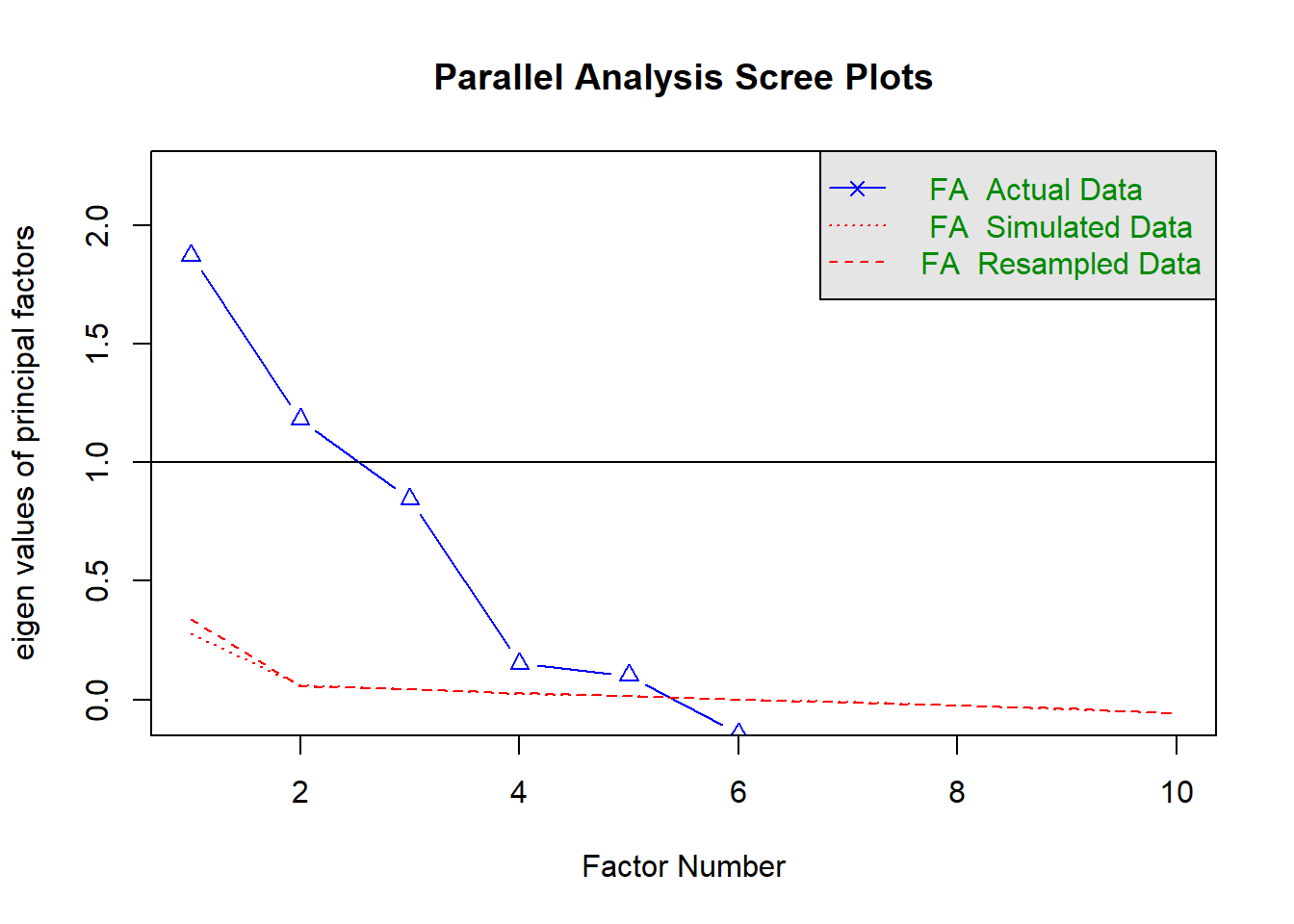Figure 1: Parallell Analysis Scree Plots

```## Parallel analysis suggests that the number of factors =  5  and the number of components =  NA
factoranalysis <- fa(tb_unique_norm, nfactors = fatest\$nfact, rotate = "oblimin", fm = "minres")
## Warning in fa.stats(r = r, f = f, phi = phi, n.obs = n.obs, np.obs = np.obs, :
## The estimated weights for the factor scores are probably incorrect. Try a
## different factor score estimation method.
## Warning in fac(r = r, nfactors = nfactors, n.obs = n.obs, rotate = rotate, : An
## ultra-Heywood case was detected. Examine the results carefully
##
##                          MR1    MR2    MR3    MR4    MR5
## salary                                 -0.979
## nremployees                      0.466
## year_n                                         0.998
## sum_pop                          0.996
## regioneduyears                   0.570
## perc_women_region         0.906
## salaryquotient
## eduquotient              -0.969
## perc_women_ssyk_region                                0.998
## occuptional...SSYK.2012.                0.779
##
##                  MR1   MR2   MR3   MR4   MR5
## Proportion Var 0.195 0.170 0.168 0.108 0.106
## Cumulative Var 0.195 0.365 0.533 0.640 0.746
##
##                          MR1    MR2    MR3    MR4    MR5
## salary                                 -0.979
## nremployees                      0.466
## year_n                                         0.998
## sum_pop                          0.996
## regioneduyears                   0.570
## perc_women_region         0.906
## salaryquotient
## eduquotient              -0.969
## perc_women_ssyk_region                                0.998
## occuptional...SSYK.2012.                0.779
##
##                  MR1   MR2   MR3   MR4   MR5
## Proportion Var 0.195 0.170 0.168 0.108 0.106
## Cumulative Var 0.195 0.365 0.533 0.640 0.746

mygrid %>%
knitr::kable(
booktabs = TRUE,
caption = 'Table of proposed exogenous variables to evaluate')```
Table 2: Table of proposed exogenous variables to evaluate
MR1 MR2 MR3 MR4 MR5
perc_women_region nremployees salary year_n perc_women_ssyk_region
eduquotient nremployees salary year_n perc_women_ssyk_region
perc_women_region sum_pop salary year_n perc_women_ssyk_region
eduquotient sum_pop salary year_n perc_women_ssyk_region
perc_women_region regioneduyears salary year_n perc_women_ssyk_region
eduquotient regioneduyears salary year_n perc_women_ssyk_region
perc_women_region nremployees occuptional…SSYK.2012. year_n perc_women_ssyk_region
eduquotient nremployees occuptional…SSYK.2012. year_n perc_women_ssyk_region
perc_women_region sum_pop occuptional…SSYK.2012. year_n perc_women_ssyk_region
eduquotient sum_pop occuptional…SSYK.2012. year_n perc_women_ssyk_region
perc_women_region regioneduyears occuptional…SSYK.2012. year_n perc_women_ssyk_region
eduquotient regioneduyears occuptional…SSYK.2012. year_n perc_women_ssyk_region

I will start by creating all possible sets and subsets from the set of five exogenous variables that were selected by the factor analysis. For each set, I will create a model from a set of structured learning algorithms from the bnlearn package. Each algorithm is evaluated against how well it minimizes the deviations from the testable implications in the model and the dataset. The function localTests from the dagitty package is used to get a numeric value of the testable implications. The mean deviation from all testable implications by localTests is used. This could favour more complex models since there are fewer testable implications in complex than in simple models. The version of localTests in my test uses a combination of categorical and continuous data. A more advanced algorithm could have been used to select the model that minimizes the deviation. The models so far are created in dagitty syntax.

From the dagitty syntax, I will create a Structural Equation Model in lavaan for each model created in the earlier step. Each SEM model is evaluated for a variety of fit measures to assess the global fit of the latent variable model. No latent variables are evaluated at this stage but you could perhaps imagine that there is a correspondence between the factor analysis and some unmeasured latent variables. The model parameters for each model is also stored for later analysis.

The table of models and the table of evaluated models are joined to allow extra comparisons.

Since the generation of dagitty models takes a while I have prepared that table in a file.

```  list_of_exogenous_variables <- allcombn(mygrid)
#table_of_dagitty_models <- combtodag(list_of_exogenous_variables, tb_unique_norm)
table_of_dagitty_models <- table_of_dagitty_models[,2:22]
table_of_sem_models <- dagitty2sem(table_of_dagitty_models, tb_unique_norm)

table_of_dagitty_models_df <- data.frame(table_of_dagitty_models)

colnames(table_of_dagitty_models_df) <-
c("model",
names(bnmodels),
c("1" , "2", "3", "4", "5",
"algorithm"))

dagitty_and_sem_table <- table_of_dagitty_models_df %>%
left_join(table_of_sem_models, by = c("1" , "2", "3", "4", "5"))
ggplot(dagitty_and_sem_table) +
geom_point(aes(x = pvalue.x, y = rmsea))```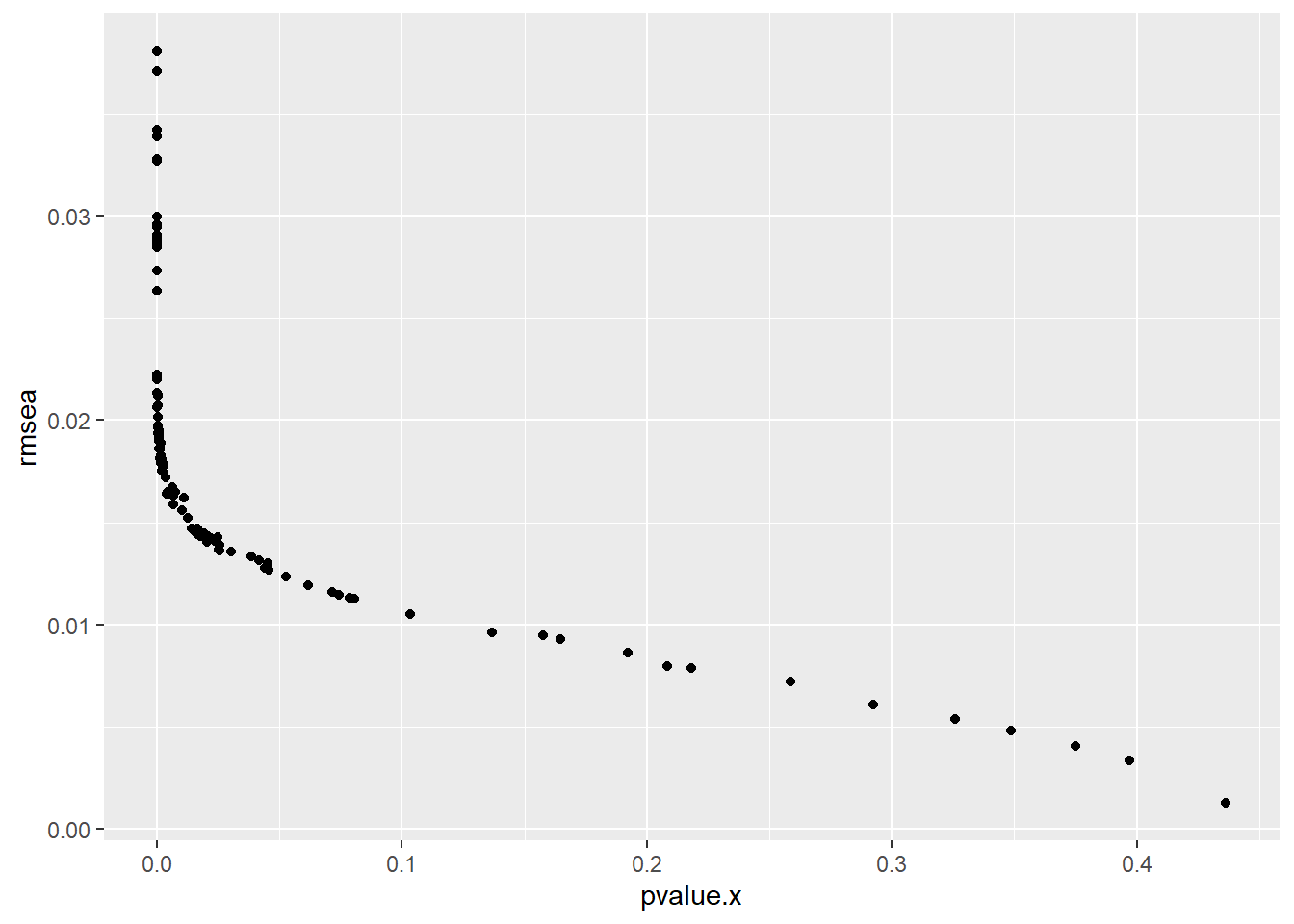Figure 2: The figure shows that there is a tradeoff between the pvalue and the Root Mean Square Error of the model

```  dagitty_and_sem_table %>%
mutate(deviance = as.numeric(pmin(h2pc, hpc, fast.iamb, inter.iamb, si.hiton.pc, iamb.fdr, iamb, gs, mmpc, pc,  hc, tabu, mmhc, rsmax2))) %>%
ggplot() +
geom_point(aes(x = aic, y = deviance))```Figure 3: The figure shows how the deviance measured by localTests and the model complexity measured in aic are related

From the table, we can examine how many of the models contained an arc, i.e. a relation from one variable to another. We find that the direction of the relation from quotient between the average number of education years for men and women to quotient between salary for men and women is found in 121 out of 143 tested models. The estimation of the relationship is 0.32 standard units. Only the models with a pvalue less than 0.05 are counted.

If we use the ten (arbitrary number, could be optimized) arcs that occur are most frequent in the models that I have analysed and use those arcs to create a whitelist, i.e. arcs that must be present in the model, when estimating a new model with the hills climbing algorithm we get a model that can be used to approximate the causal effects that can be estimated from the data. The plot shows the model. In this model year and percentage of women in the ssyk are exogenous variables and the rest of the variables are endogenous. When looking at the model’s parameter values and sorting it by the highest effect we find that the effect of per cent women in the region on quotient between the average number of education years for men and women is the highest of all effects between continuous variables, 79 out of 143 models has this direction of this relation.

```  temp <- combn(colnames(tb_unique_norm), 2)
list_of_var_combinations <- cbind(temp, rbind(temp[2,], temp[1,]))

summary_table <- vector()
for(i in data.frame(list_of_var_combinations)){
est <- dagitty_and_sem_table %>%
filter(lhs == i, rhs == i, pvalue.x < 0.05) %>%
dplyr::select(est)
summary_table <- rbind(
summary_table,
c(i,
(t(summary(est))),
nrow(est),
sd(t(est))))
}

summary_table <- unique(cbind(summary_table[,1:8], data.frame(map(data.frame(summary_table[,9:10]), as.numeric)))) %>%
arrange(-X1)

summary_table[1:10,] %>%
select(`1`, `2`, X1) %>%
rename(lhs = `1`) %>%
rename(rhs = `2`) %>%
rename(nr_of_models = X1) %>%
knitr::kable(
booktabs = TRUE,
caption = 'The ten most common arcs of all 143 models')```
Table 3: The ten most common arcs of all 143 models
lhs rhs nr_of_models
salaryquotient eduquotient 121
salaryquotient perc_women_ssyk_region 111
salaryquotient salary 110
regioneduyears year_n 110
nremployees perc_women_ssyk_region 104
salaryquotient year_n 104
salary year_n 98
occuptional…SSYK.2012. perc_women_ssyk_region 98
salaryquotient sum_pop 97
regioneduyears sum_pop 95
```  whitelist <- summary_table[1:10, 2:1]

hctree <- hc(tb_unique_norm, whitelist = whitelist)

neltree <- as.graphNEL(hctree)
if (length(bg) == 0){
return (Inf)
}

g <- dagitty(pcalg2dagitty(t(as(bg, "matrix")), colnames(tb_unique_norm), type = "dag"))

fit <- sem(paste(dagityy2lavaan(g, NULL), collapse = ''), data = tb_unique_norm)
## Warning in lav_data_full(data = data, group = group, cluster = cluster, : lavaan
## WARNING: some observed variances are (at least) a factor 1000 times larger than
## others; use varTable(fit) to investigate
semPaths(fit, 'std', 'est', curveAdjacent = TRUE, style = "lisrel")``````  r <- localTests(
g,
tb_unique_norm, "cis",
sample.cov = lavCor(tb_unique_norm),
sample.nobs = nrow( tb_unique_norm ),
max.conditioning.variables = 2,
R = 100)
## Warning in lav_data_full(data = data, group = group, cluster = cluster, : lavaan
## WARNING: some observed variances are (at least) a factor 1000 times larger than
## others; use varTable(fit) to investigate
parameterEstimates(fit) %>%
select(lhs, op, rhs, est, pvalue) %>%
arrange(-abs(est)) %>%
knitr::kable(
booktabs = TRUE,
caption = 'Parameters for the approximate model sorted in falling effect size')```
Table 3: Parameters for the approximate model sorted in falling effect size
lhs op rhs est pvalue
occuptional…SSYK.2012. ~~ occuptional…SSYK.2012. 515.4245404 0.0000000
occuptional…SSYK.2012. ~ salary -28.6174604 0.0000000
occuptional…SSYK.2012. ~ perc_women_ssyk_region -7.9191011 0.0000000
occuptional…SSYK.2012. ~ year_n 5.1547157 0.0000000
occuptional…SSYK.2012. ~ perc_women_region 2.9988570 0.0000000
occuptional…SSYK.2012. ~ sum_pop 1.7031739 0.0000006
year_n ~~ year_n 0.9997846 NA
perc_women_ssyk_region ~~ perc_women_ssyk_region 0.9997846 NA
sum_pop ~~ sum_pop 0.9770425 0.0000000
perc_women_region ~~ perc_women_region 0.9603644 0.0000000
salary ~~ salary 0.9556355 0.0000000
eduquotient ~ perc_women_region -0.9015448 0.0000000
regioneduyears ~ sum_pop 0.8082339 0.0000000
salaryquotient ~~ salaryquotient 0.7302152 0.0000000
nremployees ~~ nremployees 0.7295975 0.0000000
regioneduyears ~ eduquotient -0.6494568 0.0000000
regioneduyears ~~ regioneduyears 0.4814185 0.0000000
eduquotient ~ sum_pop 0.4493408 0.0000000
salaryquotient ~ salary -0.3776151 0.0000000
nremployees ~ sum_pop 0.3740575 0.0000000
salaryquotient ~ eduquotient -0.3242296 0.0000000
regioneduyears ~ year_n 0.3050356 0.0000000
regioneduyears ~ perc_women_region -0.2773857 0.0000000
nremployees ~ eduquotient 0.2212280 0.0000000
salary ~ perc_women_ssyk_region -0.1546639 0.0000000
salaryquotient ~ sum_pop -0.1535919 0.0000000
perc_women_region ~ sum_pop 0.1529078 0.0000000
sum_pop ~ salary 0.1508210 0.0000000
salaryquotient ~ year_n 0.1479586 0.0000000
nremployees ~ perc_women_ssyk_region 0.1465144 0.0000000
salary ~ year_n 0.1421677 0.0000000
nremployees ~ perc_women_region 0.1329411 0.0002882
eduquotient ~~ eduquotient 0.1082249 0.0000000
regioneduyears ~ salary -0.0952185 0.0000000
nremployees ~ salary -0.0951437 0.0000015
perc_women_region ~ perc_women_ssyk_region -0.0790279 0.0000001
perc_women_region ~ year_n -0.0767289 0.0000001
salaryquotient ~ perc_women_ssyk_region 0.0693828 0.0000003
perc_women_region ~ salary 0.0474522 0.0014239
eduquotient ~ year_n 0.0283044 0.0000000
regioneduyears ~~ salaryquotient -0.0201865 0.0204311
nremployees ~~ salaryquotient -0.0040980 0.7020812
nremployees ~ occuptional…SSYK.2012. 0.0037042 0.0000000
salaryquotient ~ occuptional…SSYK.2012. -0.0032636 0.0000000
regioneduyears ~ occuptional…SSYK.2012. -0.0029127 0.0000000
nremployees ~~ regioneduyears -0.0017782 0.8380207
perc_women_ssyk_region ~~ year_n -0.0005915 NA
`  plotLocalTestResults( r )`Figure 4: This figure shows the testable implications from localTests

```  ggplot(data = tb_unique_norm, mapping = aes(x = eduquotient, y = salaryquotient)) +
geom_boxplot(mapping = aes(group = cut_width(eduquotient, 0.4)))```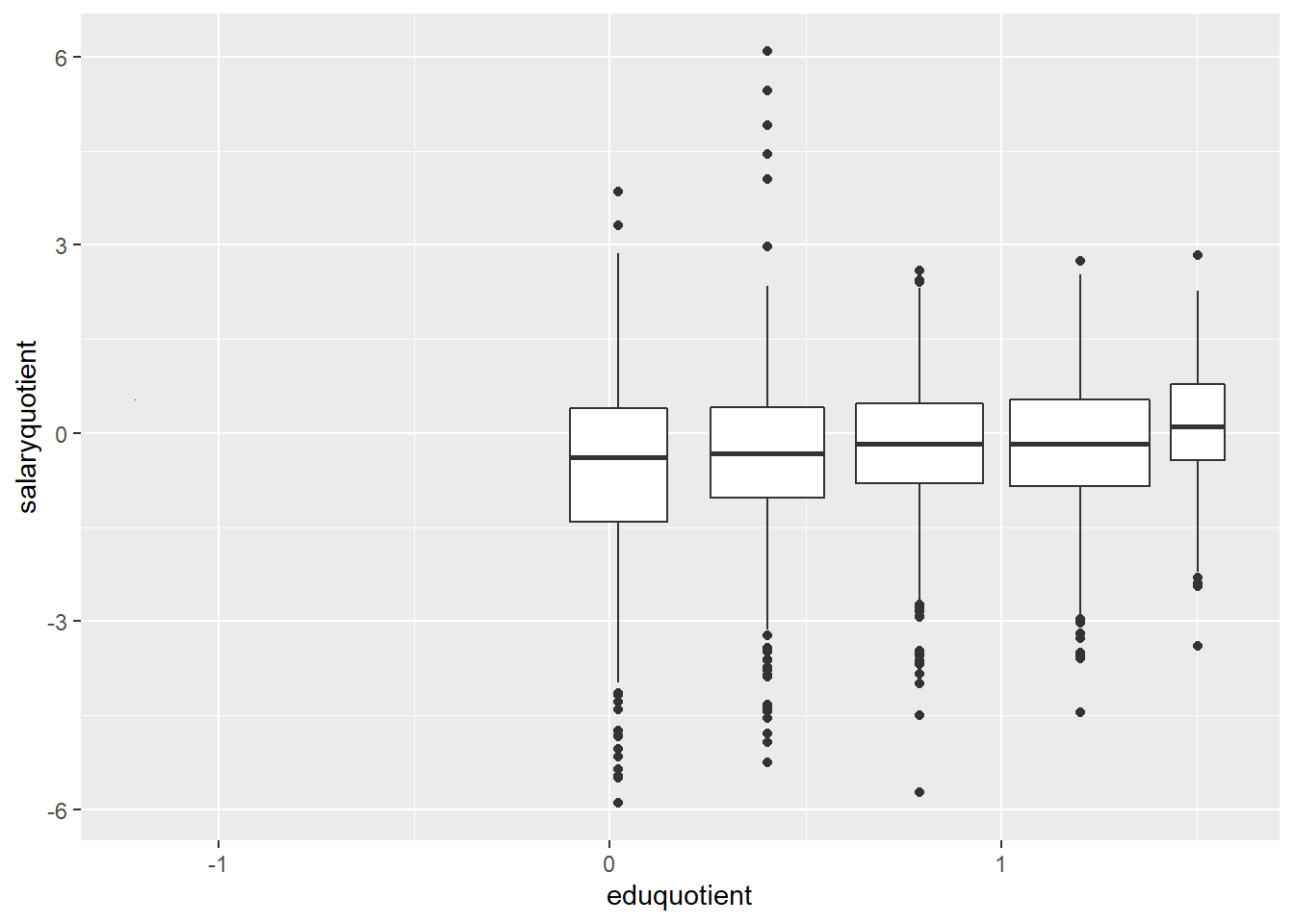Figure 5: The figure shows how the quotient between salary for men and women is affected by the quotient between the average number of education years for men and women

```  ggplot(tb_unique_norm) +
geom_point(aes(x = perc_women_region, y = eduquotient))```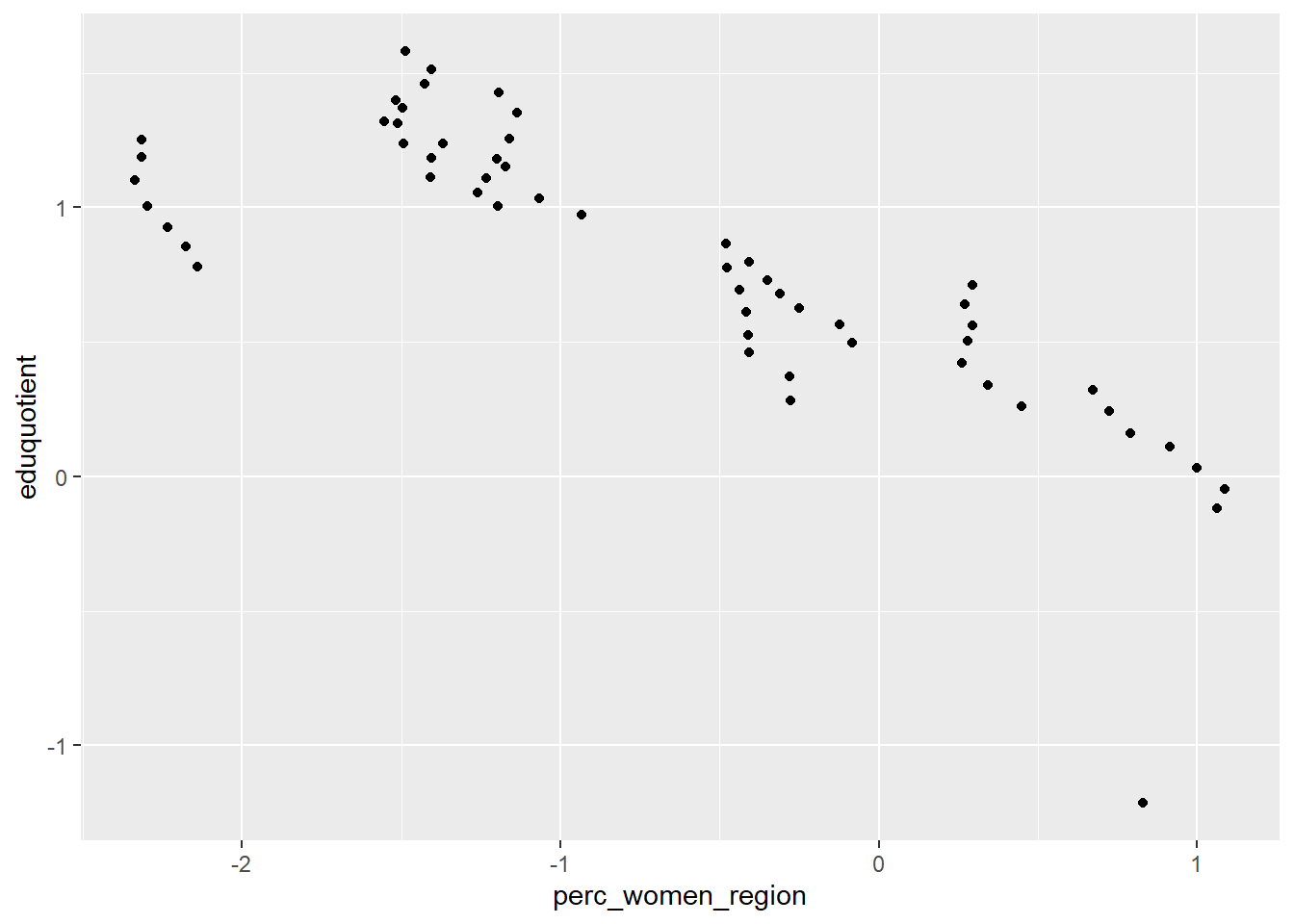Figure 6: The figure shows how the quotient between the average number of education years for men and women is affected by the per cent women in the region

If you know the DAG it is possible to identify the sets of covariates that allow unbiased estimation of causal effects with the function adjustmentSets in the dagitty package. I will calculate the possible adjustment sets for all models created in earlier steps and compare them. Let’s start by calculating the causal effect of the quotient between the average number of education years for men and women on the quotient between salary for men and women.

```  causaleffect <- calceffect(table_of_dagitty_models, "eduquotient", "salaryquotient", tb_unique_norm)

causaleffect_df <- data.frame(map(causaleffect, unlist))

colnames(causaleffect_df) <- colnames(causaleffect)

dagitty_sem_and_causal_table <- dagitty_and_sem_table %>%
left_join(causaleffect_df, by = c("1" , "2", "3", "4", "5"))
dagitty_sem_and_causal_table %>%
filter(lhs == "salaryquotient", rhs == "eduquotient") %>%
ggplot() +
geom_point(aes(x = estimate, y = pvalue.x, color = aic))```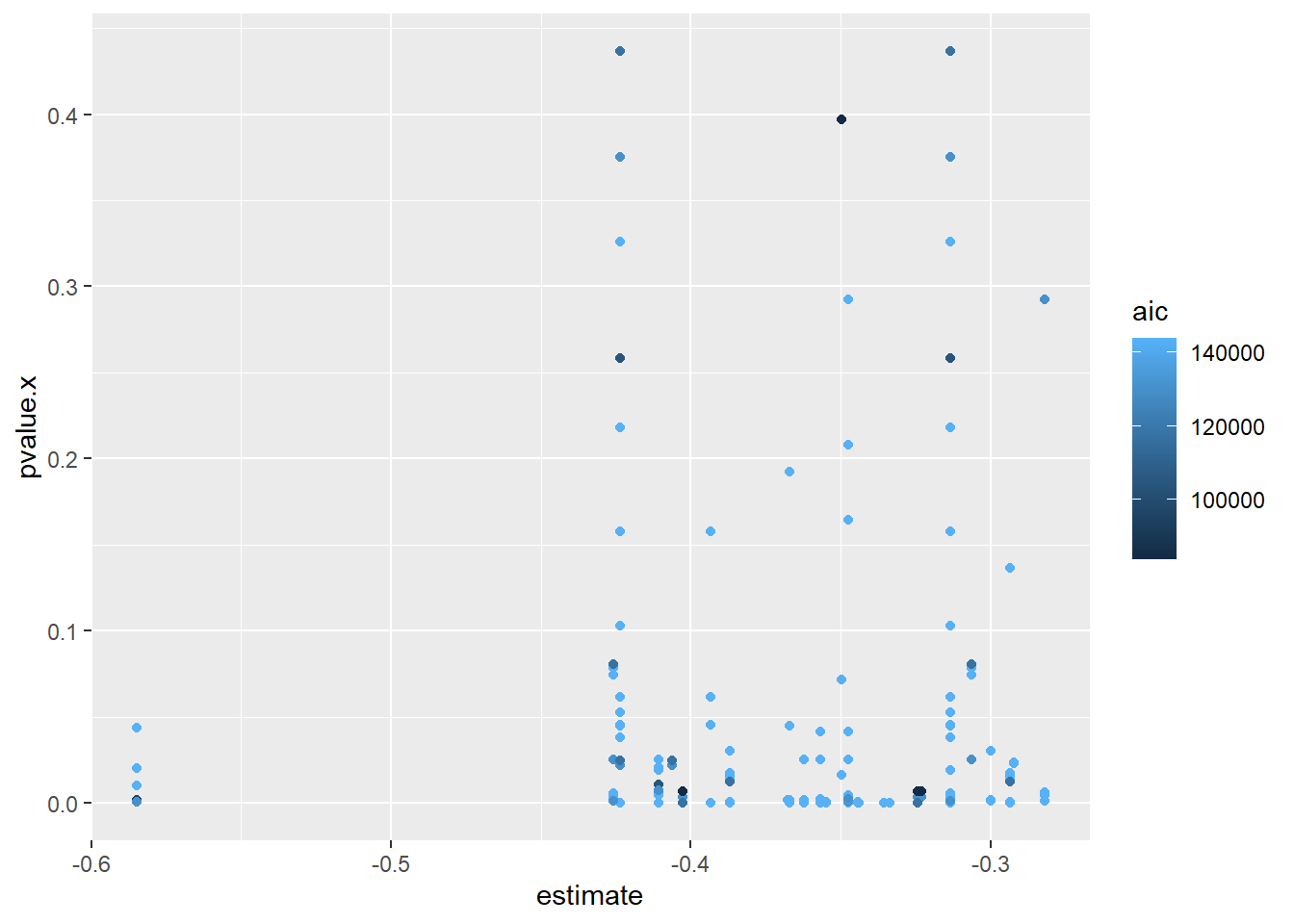Figure 7: The figure shows the estimate for the causal effect of the quotient between the average number of education years for men and women on quotient between salary for men and women from all tested models and the pvalue and aic of the model

```  dagitty_sem_and_causal_table %>%
mutate(modelnumber = as.integer(factor(`t(data.frame(eq))`))) %>%
filter(lhs == "salaryquotient", rhs == "eduquotient") %>%
ggplot() +
geom_point(aes(x = modelnumber, y = estimate, color = pvalue.x))```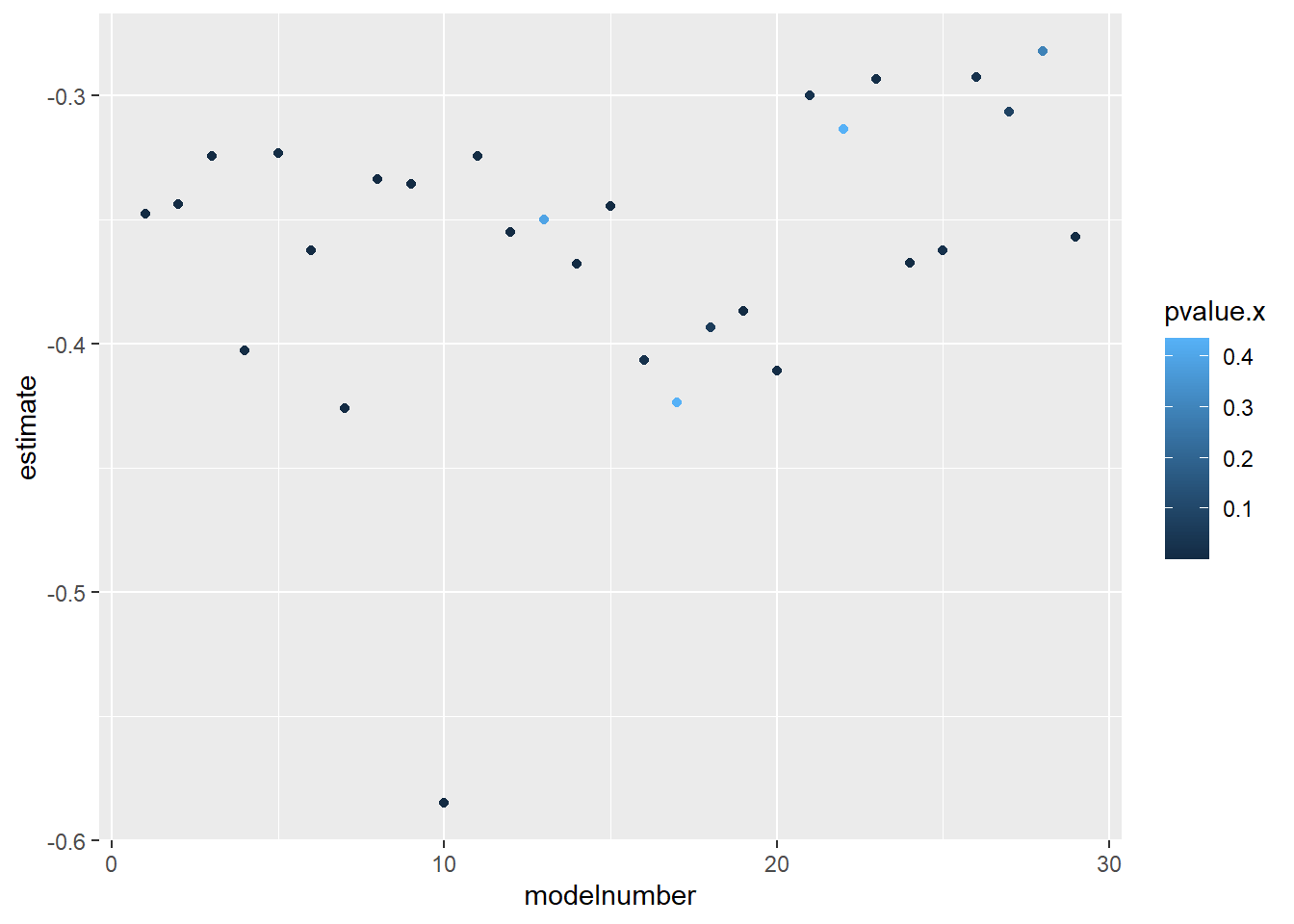Figure 8: The figure shows how the quotient between the average number of education years for men and women is affected by the per cent women in the region for the different covariates

```  dagitty_sem_and_causal_table %>%
filter(lhs == "salaryquotient", rhs == "eduquotient") %>%
mutate(modelnumber = as.integer(factor(`t(data.frame(eq))`))) %>%
mutate(linear_equation_with_covariates = `t(data.frame(eq))`) %>%
group_by(modelnumber) %>%
mutate(frequency = n()) %>%
arrange(modelnumber) %>%
select(linear_equation_with_covariates, modelnumber, estimate, frequency) %>%
unique() %>%
knitr::kable(
booktabs = TRUE,
caption = 'Table showing the covariates needed for different models to calculate the effect')```
Table 4: Table showing the covariates needed for different models to calculate the effect
linear_equation_with_covariates modelnumber estimate frequency
salaryquotient ~ eduquotient 1 -0.3475676 13
salaryquotient ~ eduquotient + nremployees 2 -0.3438332 5
salaryquotient ~ eduquotient + nremployees + occuptional…SSYK.2012. 3 -0.3242904 1
salaryquotient ~ eduquotient + nremployees + occuptional…SSYK.2012. + perc_women_region + sum_pop + year_n 4 -0.4027556 31
salaryquotient ~ eduquotient + nremployees + occuptional…SSYK.2012. + salary + sum_pop + year_n 5 -0.3230239 7
salaryquotient ~ eduquotient + nremployees + perc_women_region + salary + sum_pop 6 -0.3621844 2
salaryquotient ~ eduquotient + nremployees + perc_women_region + sum_pop + year_n 7 -0.4259852 10
salaryquotient ~ eduquotient + nremployees + perc_women_ssyk_region + salary 8 -0.3335892 1
salaryquotient ~ eduquotient + occuptional…SSYK.2012. 9 -0.3355981 1
salaryquotient ~ eduquotient + occuptional…SSYK.2012. + perc_women_region + regioneduyears 10 -0.5848968 7
salaryquotient ~ eduquotient + occuptional…SSYK.2012. + perc_women_ssyk_region + salary + sum_pop + year_n 11 -0.3242342 31
salaryquotient ~ eduquotient + occuptional…SSYK.2012. + perc_women_ssyk_region + year_n 12 -0.3548155 2
salaryquotient ~ eduquotient + occuptional…SSYK.2012. + regioneduyears 13 -0.3497428 3
salaryquotient ~ eduquotient + occuptional…SSYK.2012. + regioneduyears + year_n 14 -0.3676810 1
salaryquotient ~ eduquotient + occuptional…SSYK.2012. + year_n 15 -0.3443816 3
salaryquotient ~ eduquotient + perc_women_region + perc_women_ssyk_region + sum_pop + year_n 16 -0.4063135 2
salaryquotient ~ eduquotient + perc_women_region + regioneduyears + sum_pop + year_n 17 -0.4235753 17
salaryquotient ~ eduquotient + perc_women_region + salary + sum_pop + year_n 18 -0.3932932 4
salaryquotient ~ eduquotient + perc_women_region + sum_pop 19 -0.3868834 11
salaryquotient ~ eduquotient + perc_women_region + sum_pop + year_n 20 -0.4106547 11
salaryquotient ~ eduquotient + perc_women_ssyk_region + salary + sum_pop 21 -0.2998376 3
salaryquotient ~ eduquotient + perc_women_ssyk_region + salary + sum_pop + year_n 22 -0.3135959 23
salaryquotient ~ eduquotient + perc_women_ssyk_region + sum_pop 23 -0.2934366 10
salaryquotient ~ eduquotient + perc_women_ssyk_region + year_n 24 -0.3671626 5
salaryquotient ~ eduquotient + regioneduyears 25 -0.3622676 2
salaryquotient ~ eduquotient + salary + sum_pop 26 -0.2923565 2
salaryquotient ~ eduquotient + salary + sum_pop + year_n 27 -0.3064032 4
salaryquotient ~ eduquotient + sum_pop 28 -0.2821068 4
salaryquotient ~ eduquotient + year_n 29 -0.3567006 5
```  ggplot(tb_unique_norm) +
geom_point(aes(x = eduquotient, y = salaryquotient, color = perc_women_region))```Figure 9: The figure shows how quotient between salary for men and women is affected by quotient between the average number of education years for men and women and the covariate per cent women in the region

As a second example, I will calculate the causal effect of per cent women in the region on quotient between the average number of education years for men and women.

```  causaleffect <- calceffect(table_of_dagitty_models, "perc_women_region", "eduquotient", tb_unique_norm)

causaleffect_df <- data.frame(map(causaleffect, unlist))

colnames(causaleffect_df) <- colnames(causaleffect)

dagitty_sem_and_causal_table <- dagitty_and_sem_table %>%
left_join(causaleffect_df, by = c("1" , "2", "3", "4", "5"))
dagitty_sem_and_causal_table %>%
filter(lhs == "eduquotient", rhs == "perc_women_region") %>%
ggplot() +
geom_point(aes(x = estimate, y = pvalue.x, color = aic))```Figure 10: The figure shows the estimate for the causal effect of per cent women in the region on quotient between the average number of education years for men and women

```  dagitty_sem_and_causal_table %>%
mutate(modelnumber = as.integer(factor(`t(data.frame(eq))`))) %>%
filter(lhs == "eduquotient", rhs == "perc_women_region") %>%
ggplot() +
geom_point(aes(x = modelnumber, y = estimate, color = aic))```Figure 11: The figure shows how the quotient between the average number of education years for men and women is affected by the per cent women in the region for the different covariates